Test: Signal Generators & Waveform Shaping Circuit

# Test: Signal Generators & Waveform Shaping Circuit

Test Description

## 10 Questions MCQ Test Topicwise Question Bank for Electronics Engineering | Test: Signal Generators & Waveform Shaping Circuit

Test: Signal Generators & Waveform Shaping Circuit for Electronics and Communication Engineering (ECE) 2023 is part of Topicwise Question Bank for Electronics Engineering preparation. The Test: Signal Generators & Waveform Shaping Circuit questions and answers have been prepared according to the Electronics and Communication Engineering (ECE) exam syllabus.The Test: Signal Generators & Waveform Shaping Circuit MCQs are made for Electronics and Communication Engineering (ECE) 2023 Exam. Find important definitions, questions, notes, meanings, examples, exercises, MCQs and online tests for Test: Signal Generators & Waveform Shaping Circuit below.
Solutions of Test: Signal Generators & Waveform Shaping Circuit questions in English are available as part of our Topicwise Question Bank for Electronics Engineering for Electronics and Communication Engineering (ECE) & Test: Signal Generators & Waveform Shaping Circuit solutions in Hindi for Topicwise Question Bank for Electronics Engineering course. Download more important topics, notes, lectures and mock test series for Electronics and Communication Engineering (ECE) Exam by signing up for free. Attempt Test: Signal Generators & Waveform Shaping Circuit | 10 questions in 30 minutes | Mock test for Electronics and Communication Engineering (ECE) preparation | Free important questions MCQ to study Topicwise Question Bank for Electronics Engineering for Electronics and Communication Engineering (ECE) Exam | Download free PDF with solutions
 1 Crore+ students have signed up on EduRev. Have you?
Test: Signal Generators & Waveform Shaping Circuit - Question 1

### Hysteresis is desirable in Schmitt-trigger because

Detailed Solution for Test: Signal Generators & Waveform Shaping Circuit - Question 1

Hysteresis is desirable in a Schmitt trigger because it prevents noise from causing false triggering.

Test: Signal Generators & Waveform Shaping Circuit - Question 2

### Assertion (A): All oscillators require positive feedback for their operation. Reason (R): Any circuit which is used to generate a periodic voltage without an a.c. input signal is called an oscillator.

Detailed Solution for Test: Signal Generators & Waveform Shaping Circuit - Question 2

Ail oscillators do not require positive feedback for their operation because if the positive resistance of the LC tank circuit is cancelled by introducing the right amount of negative resistance across the tank circuit, then the steady oscillation can be maintained. Some of the devices which possess negative resistance as mentioned above are dynatron, transitron, UJT and tunnel diode. Hence, assertion is not correct.

Test: Signal Generators & Waveform Shaping Circuit - Question 3

### Each stage of a three stage amplifier without feedback has identical pole frequencies and its open loop transfer function is expressed as: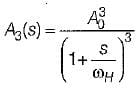What is the maximum value of feedback factor βmax  for which the amplifier remains stable?

Detailed Solution for Test: Signal Generators & Waveform Shaping Circuit - Question 3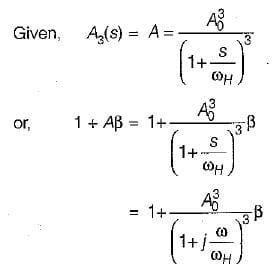The phase change may change Aβ to negative. This can happen only when the total change in the phase angle of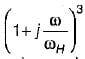becomes 1800 . The  required phase change in one stage i.e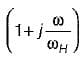should be 600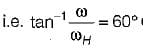or,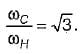∴ Here, ωc is the frequency at which the phase angle changes the sign. The value of Aβ at this frequency is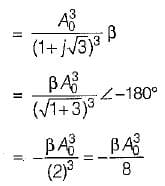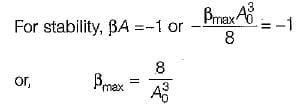Test: Signal Generators & Waveform Shaping Circuit - Question 4

Assertion (A): In a crystal oscillator, it is necessary to enclose the crystal in a temperature controlled oven to achieve the frequency stability of the order of 1 partin 1010.
Reason (R): A crystal has very high Q as a resonant circuit, which results in good frequency stability for the oscillator.

Detailed Solution for Test: Signal Generators & Waveform Shaping Circuit - Question 4

Both assertion and reason are individually correct statements. The correct reason for the assertion is that the resonant frequency of the crystal is temperature dependent, therefore it is necessary to enclose the crystal in a temperature controlled oven to achieve the required frequeny stability.

Test: Signal Generators & Waveform Shaping Circuit - Question 5

Assertion (A): If the magnitude of loop gain of the amplifier is unity then the amplifier gives an a.c. output without any a.c. input signal.
Reason (R): The property of the regenerative feedback is utilised in oscillators.

Detailed Solution for Test: Signal Generators & Waveform Shaping Circuit - Question 5

The closed-iooD aain with positive feedback is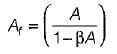If the magnitude of loop gain i.e. |βA| - 1, then Af = ∞. Hence, the gain of the amplifier with positive feedback is infinite and the amplifier gives an a.c. output without any a.c. input signal. Thus, the amplifier acts as an oscillator. Regenerative or positive feedback is utilized in oscillators.
Hence, both assertion and reason are correct but reason is not the correct explanation of assertion.

Test: Signal Generators & Waveform Shaping Circuit - Question 6

In a Wien-bridge oscillator, if the value of R is 100 kΩ, and frequency of oscillation is 10 kHz, then the value of capacitance C would be

Detailed Solution for Test: Signal Generators & Waveform Shaping Circuit - Question 6

The operating frequency of a Wien-bridge oscillator is given by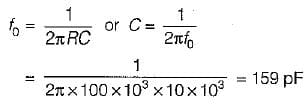Test: Signal Generators & Waveform Shaping Circuit - Question 7

A 555 time basically operates in

Test: Signal Generators & Waveform Shaping Circuit - Question 8

For an RC phase-shift oscillator using BJT, R = 10 kΩ, C = 0.01 μ.F and Rc = 2.2 kΩ The minimum current gain needed for sustained oscillations is around

Detailed Solution for Test: Signal Generators & Waveform Shaping Circuit - Question 8

For sustained oscillations, the minimum vaiue of current gain or forward current gain is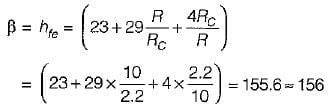Test: Signal Generators & Waveform Shaping Circuit - Question 9

A tank circuit contains an inductance of 1 mH. What is the range of tuning capacitor value if the resonant frequency ranges from (540-1650) kHz?

Detailed Solution for Test: Signal Generators & Waveform Shaping Circuit - Question 9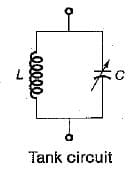For the given tank circuit,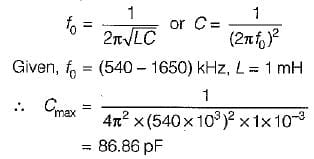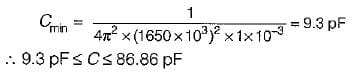Test: Signal Generators & Waveform Shaping Circuit - Question 10

ln the Hartley oscillator, L2 = 0.4 mH and C - 0.004 μF. If the frequency of the oscillator is 120 kHz, then the value of L1 would be (neglect the mutual inductance)

Detailed Solution for Test: Signal Generators & Waveform Shaping Circuit - Question 10

The frequency of Hartley oscillator is given by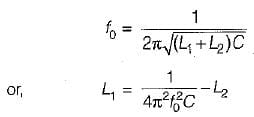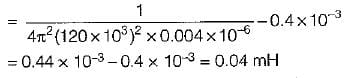## Topicwise Question Bank for Electronics Engineering

193 tests
Information about Test: Signal Generators & Waveform Shaping Circuit Page
In this test you can find the Exam questions for Test: Signal Generators & Waveform Shaping Circuit solved & explained in the simplest way possible. Besides giving Questions and answers for Test: Signal Generators & Waveform Shaping Circuit, EduRev gives you an ample number of Online tests for practice

## Topicwise Question Bank for Electronics Engineering

193 tests(Scan QR code)